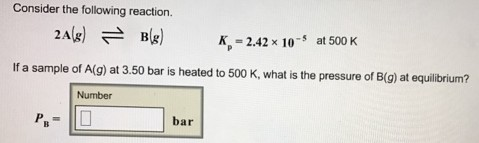# Problem: Consider the following reaction. 2A(g) ⇌ B(g)    Kp = 2.42 x 10 -5 at 500 KIf a sample of A(g) at 3.50 bar is heated to 500 K, what is the pressure of B(g) at equilibrium?PB =

###### FREE Expert Solution
91% (217 ratings)###### Problem Details

Consider the following reaction.

2A(g) ⇌ B(g)    Kp = 2.42 x 10 -5 at 500 K

If a sample of A(g) at 3.50 bar is heated to 500 K, what is the pressure of B(g) at equilibrium?

PB =